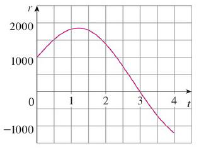Chapter 5.4, Problem 68E### Single Variable Calculus: Early Tr...

8th Edition
James Stewart
ISBN: 9781305270343

#### Solutions

Chapter
Section### Single Variable Calculus: Early Tr...

8th Edition
James Stewart
ISBN: 9781305270343
Textbook Problem

# Water flows into and out of a storage tank. A graph of the rate of change r(t) of the volume of water in the tank, in liters per day, is shown. If the amount of water in the tank at time t = 0 is 25,000 L, use the Midpoint Rule to estimate the amount of water in the tank four days later.To determine

To calculate: The amount of water in the tank after the 4 days duration by using the Midpoint Rule.

Explanation

Given information:

The amount of water in the tank at time t=0 is r(0)=25,000 L.

The general expression for Midpoint Rule is shown below:

abf(x)dxi=1nf(x¯i)Δx=Δx(f(x¯1)+.......+f(x¯n))

Apply the conditions for midpoint rule as follows:

Δx=ban (1)

x¯i=12(xi1+xi) (2)

Here, width of the subinterval is Δx, the upper limit is b, the lower limit is a, the number of subintervals is n, and the midpoint of (xi1,xi) is x¯i.

Calculation:

Find the width of the subinterval (Δt) using Equation (1) as shown below.

Substitute 4 for n, 4 days for b, and 0 for a in Equation (1).

Δt=404=1day

The expression to find the amount of water in the tank for four days using midpoint rule is shown below.

04r(t)dtΔt(r(t¯1)+r(t¯2)+r(t¯3)+r(t¯4)) (3)

Here, the amount of water at time t¯1 is r(t¯1), the amount of water at time t¯2 is r(t¯2), the amount of water at time t¯3 is r(t¯3), and the amount of water at time t¯4 is r(t¯4)

### Still sussing out bartleby?

Check out a sample textbook solution.

See a sample solution

#### The Solution to Your Study Problems

Bartleby provides explanations to thousands of textbook problems written by our experts, many with advanced degrees!

Get Started

#### Find more solutions based on key concepts